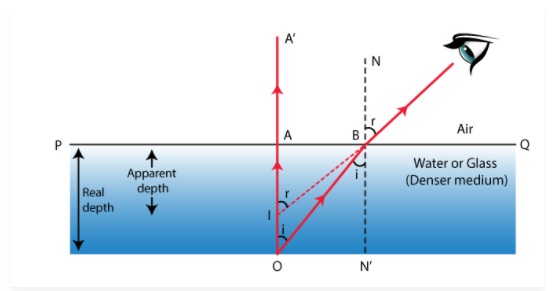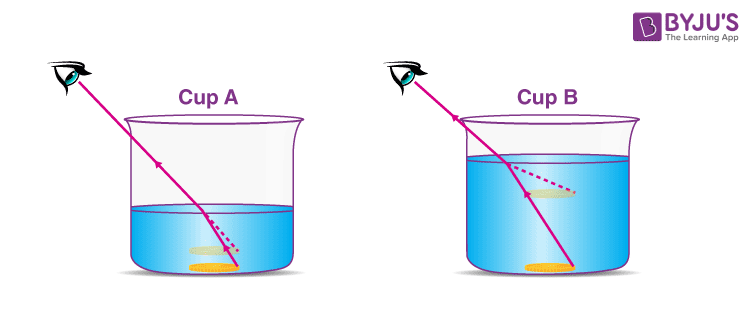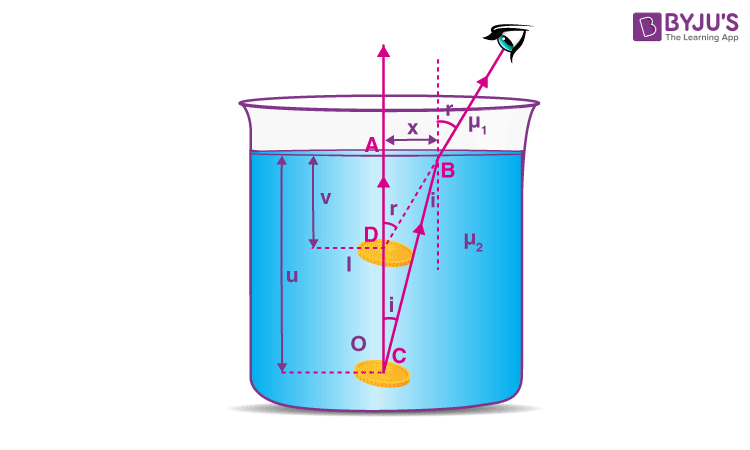Know more about the Repeaters Batch Know more about the Repeaters Batch

# Apparent Depth

When we look into a tank filled with water, the bottom of the tank will appear to be raised. The real depth is the actual depth of the bottom of the tank and the apparent depth is the virtual depth that is observed as a result of the refraction of light. The apparent depth depends upon the refractive index of the medium. The refractive index is a measure of the bending of the ray as it passes from one medium to another. It is given by the ratio of the sine of the angle of incidence to the sine of the angle of refraction. The refractive index can also be defined in terms of the velocity of light. The ratio of the velocity of light in the vacuum to the velocity of light in the given medium is also called the refractive index.The refraction of light through the atmosphere is responsible for many interesting phenomena. Due to the refraction of light, the sun can be seen a little earlier than the actual sunrise and a little later than the actual sunset. By actual sunrise, we mean the sun crossing the horizon. The refractive index of air w.r.t to vacuum is 1.00029. Therefore, the apparent shift in the position of the sun is about half a degree and the time difference between actual and apparent sunrise is about 2 minutes. The change in the shape of the sun to an elliptical shape just before setting is also due to the refraction of light.

## Definition of Real Depth and Apparent Depth

### Real Depth

The actual depth of an object beneath the surface is called the Real Depth. For example, if a coin is immersed in a beaker of water, the real depth of the coin from the surface of the water can be found using a measuring scale.

### Apparent Depth

If an observer looks at a coin immersed in a beaker of water (denser medium) from the air (rarer medium), the depth of the coin seems a little elevated. The distance from the surface of the water to the elevated point is called the apparent depth. The apparent depth is smaller than the real depth.

The apparent depth also depends on the depth of water in the beaker. Consider two beakers, one partially filled with water and the other fully filled with water. A similar coin is immersed in both the beakers. When the two beakers are viewed from the same position, the coin will be seen in the beaker fully filled with water and the coin cannot be seen in the beaker partially filled with water. The reason for this is that the apparent depth changes when the level of water in the beaker is increased and decreased.## How is the apparent depth and the real depth related to the refractive index?Consider a beaker filled with water and a coin is placed at the bottom of the beaker. Let μ2 be the refractive index of water and μ1 be the refractive index of air. The real depth of the coin is taken as u, u is the distance of the coin from the free surface. The coin is observed from a point in the air. Two different rays are considered in order to determine the apparent depth. One of the rays is taken along the normal and the second ray is taken at an angle from the normal. As the ray travels from the denser medium water to the rarer medium air, it will bend away from the normal. The figure below shows the formation of the virtual image of the coin due to the refraction of light. The distance from the free surface to the virtual image is called the apparent depth. The apparent depth is marked as v.

The angle of incidence and the angle of refraction is marked. Since the interior alternate angles for parallel lines are going to be the same, the other angles also can be determined. Now, applying Snell’s law we have

μ2 sin i = μ1 sin r ——-(1)

The rays are paraxial, a paraxial ray is a ray that makes very small angles. Since the angles are small, we can consider that Sin θ ≈ θ ≈ tan θ. Therefore, Snell’s law can be written in terms of tan θ. Hence, equation (1) becomes

μ2 tan i = μ1 tan r ——(2)

Considering the triangle ABC, we get tan i = x/u.

Considering the smaller triangle ABD, we get tan r = x/v.

Substituting the values of tan i and tan r in equation (2) we have

μ2 (x/u) = μ1 (x/v)

Therefore,

$$\begin{array}{l}\frac{\mu _{2}}{\mu _{1}}=\frac{u}{v}\end{array}$$

Here, u is the real depth

v is the apparent depth

μ1 is the refractive index of air

μ2 is the refractive index of water

### Solved Problems

1. A coin is placed at the bottom of a beaker filled with water up to a height of 10 cm. The refractive index of water w.r.t air is 4/3. Find the apparent depth of the coin.

Solution:

Real depth = 10 cm

Refractive index of water w.r.t air = 4/3

$$\begin{array}{l}\frac{\mu _{2}}{\mu _{1}}=\frac{real\: depth}{apparent \: depth}\end{array}$$

4/3 = 10/apparent depth

⇒ Apparent depth = 10 x (¾)

= 7.5 cm

2. Just before setting, the sun appears to be elliptical. This happens because of

a.Diffraction

b. Refraction

c. Reflection

d. Interference

#### Real and Apparent Depth Video Lesson### Apparent Depth## Frequently Asked Questions on Apparent Depth

### What is the cause for apparent depth?

The apparent depth is caused due to the refraction of light as the light rays travel from one medium to another.

### How are the real depth and apparent depth related to the refractive index?

Refractive index = Real depth/Apparent depth

### What is the apparent position of an object?

The position of the object in space as seen by the observer is called the apparent position of an object.

### What is the normal shift?

When the object is in one medium and viewed by an observer along the normal from a different medium, the image of the object appears to be shifted from the original position. This shift in the position is called the normal shift.